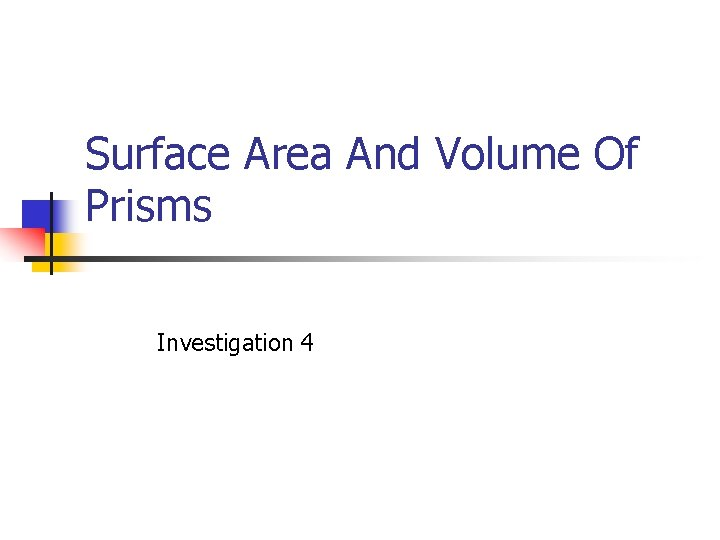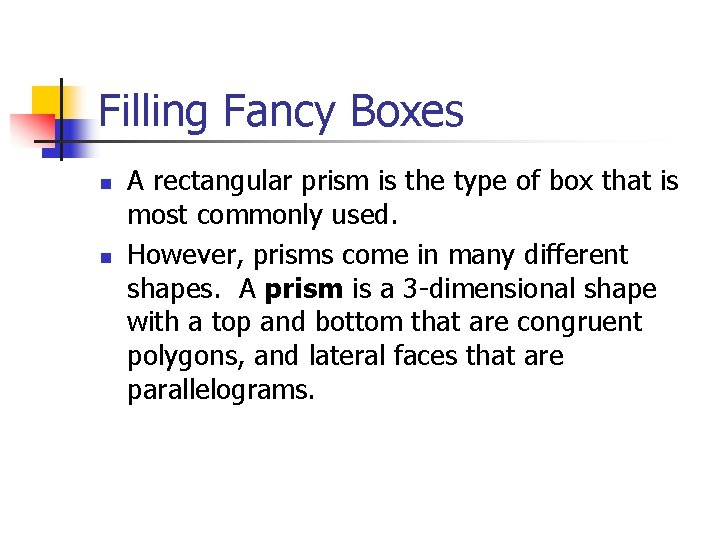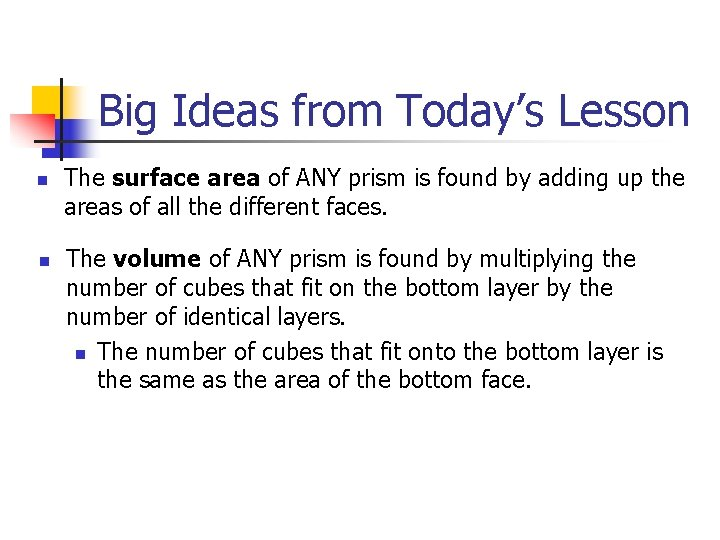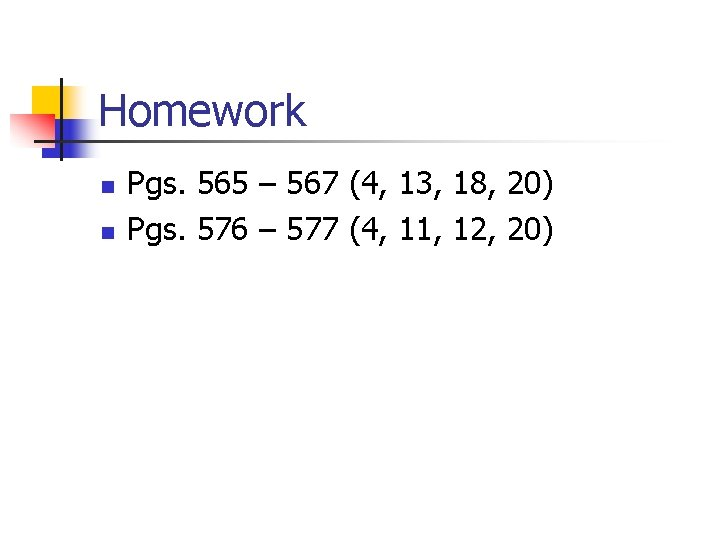# Surface Area And Volume Of Prisms Investigation 4

• Slides: 9Surface Area And Volume Of Prisms Investigation 4Today’s Learning Goal n n We will continue to work with flat patterns of prisms to help us see the total area of the 3 -D shape. We will continue to think about how to find the volume of prisms. Today we will look at non-rectangular prisms.Filling Fancy Boxes n n A rectangular prism is the type of box that is most commonly used. However, prisms come in many different shapes. A prism is a 3 -dimensional shape with a top and bottom that are congruent polygons, and lateral faces that are parallelograms.Filling Fancy Boxes n n A prism is named for the shape of its base. For example, the base of a rectangular prism is a rectangle (hence its name). Can you name the prisms below? Triangular prism Square prism Pentagonal prism Hexagonal prismFilling Fancy Boxes n n You have seen that you can find the surface area of a rectangular prism by finding the area of each of its sides and adding those areas together. Also, you have seen that you can find the volume of a rectangular prism by thinking about the number of unit cubes that would fit inside the prism.Partner Work n n Do you think similar ideas will work for the surface area and volumes of other prisms? You have 20 minutes to work on the following questions with your partner.For those that finish early The drawing below shows a prism with an odd-shaped top and bottom and rectangular sides. The top and bottom each have an area of 10 cm 2, and the height is 4 cm. a) What is the volume of the prism? b) Explain how you found the volume and why you think your method works. c) Explain how you would find the surface area of the prism.Big Ideas from Today’s Lesson n n The surface area of ANY prism is found by adding up the areas of all the different faces. The volume of ANY prism is found by multiplying the number of cubes that fit on the bottom layer by the number of identical layers. n The number of cubes that fit onto the bottom layer is the same as the area of the bottom face.Homework n n Pgs. 565 – 567 (4, 13, 18, 20) Pgs. 576 – 577 (4, 11, 12, 20)